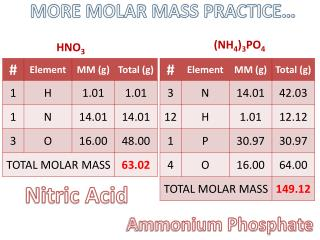DownloadDownload PresentationHNO 3

# HNO 3

Download Presentation## HNO 3

- - - - - - - - - - - - - - - - - - - - - - - - - - - E N D - - - - - - - - - - - - - - - - - - - - - - - - - - -
##### Presentation Transcript

1. MORE MOLAR MASS PRACTICE… (NH4)3PO4 HNO3 Nitric Acid Ammonium Phosphate

2. g element x 100 mass % of element = molar mass of compound : : : : percentage composition: the mass % of each element in a compound Find the % composition of ammonium phosphate. (NH4)3PO4 42.03 g N = 28.18% N 149.12 g 12.12 g H 149.12 g = 8.13% H 30.97 g P = 20.77% P 149.12 g = 42.92% O 64.00 g O 149.12 g

3. Different Types of Formulas C6H6 • Molecular Formula – shows # of atoms in one molecule or formula unit • Empirical Formula – shows smallest whole number mole ratio • Structural Formula- molecular formula info PLUS bonding electrons and atomic arrangement CH All different types of formulas for the same compound…benzene!

4. Finding an Empirical Formula from Experimental Data 1. Find mass of each element (grams) 2. Find mols of each element (convert from grams to mols) 3. Divide each “# of mol” by the smallest “# of mol” (ratios) 4. Write out formula with ratios from step 3 as subscripts (multiply by smallest integer possible for whole #’s if needed) PRACTICE... A compound is 45.5% yttrium and 54.5% chlorine. Find its empirical formula. YCl3

5. A ruthenium/sulfur compound is 67.7% Ru. Find its empirical formula. RuS1.5 Ru2S3

6. (Empirical Formula)n = Molecular Formula To find molecular formula… A. Find empirical formula. B. Find mass of empirical formula. C. Find n = Molar Mass molecular empirical mass D. Multiply all parts of empirical formula by n. “How many empiricals fit into the molecular?”

7. A carbon/hydrogen compound is 7.7% H and has a molar mass of 78 g. Find its molecular formula. emprical formula = CH Empirical mass = 78 g n = C6H6 13 g = 6 13 g

8. 92 g 46 g A compound has 26.33 g nitrogen, 60.20 g oxygen, and molar mass 92 g. Find molecular formula. ÷ 1.879 ÷ 1.879 Empirical formula = NO2 N2O4 MMempirical = = 2 n = 46 g

9. Review Problems A 17.40 g sample of a technetium/oxygen compound contains 11.07 g of Tc. Find the empirical formula. TcO3.5 Tc2O7

10. Review Problems A compound contains 70.35 g C and 14.65 g H. Its molar mass is 58 g. Find its molecular formula. emp. form.  C2H5 58 g n = C4H10 MMemp= 29 g = 2 29 g

11. Review Problems A compound contains 4.63 g lead, 1.25 g nitrogen, and 2.87 g oxygen. Name the compound. PbN4O8 ? ? lead (IV)nitrite Pb(NO2)4 (plumbicnitrite) Pb? 4 NO21–

12. LET’S REVIEW… # of H2O molecules # of H atoms # of O atoms 1 2 3 100 6.02 x 1023 2 1 4 2 6 3 200 100 2 (6.02 x 1023)‏ 6.02 x 1023 molar mass: the mass of one mole of a substance 18.0 g 2.0 g 16.0 g

13. part whole % = x 100 % QUICK SUMMARY 6.022x1023 Molar Mass vs. Atomic Mass 2 g H2 = _____ H2 = _______ 2 amu 18 g H2O = _____ H2O = ________ 18 amu 120 g MgSO4 = _____ MgSO4 = ________ 120 amu 149 g (NH4)3PO4 = _____ (NH4)3PO4 = ________ 149 amu Percentage Composition Empirical Formula • %  g • g  mol • mol • mol Empirical vs. Molecular Formula (lowest ratio)‏